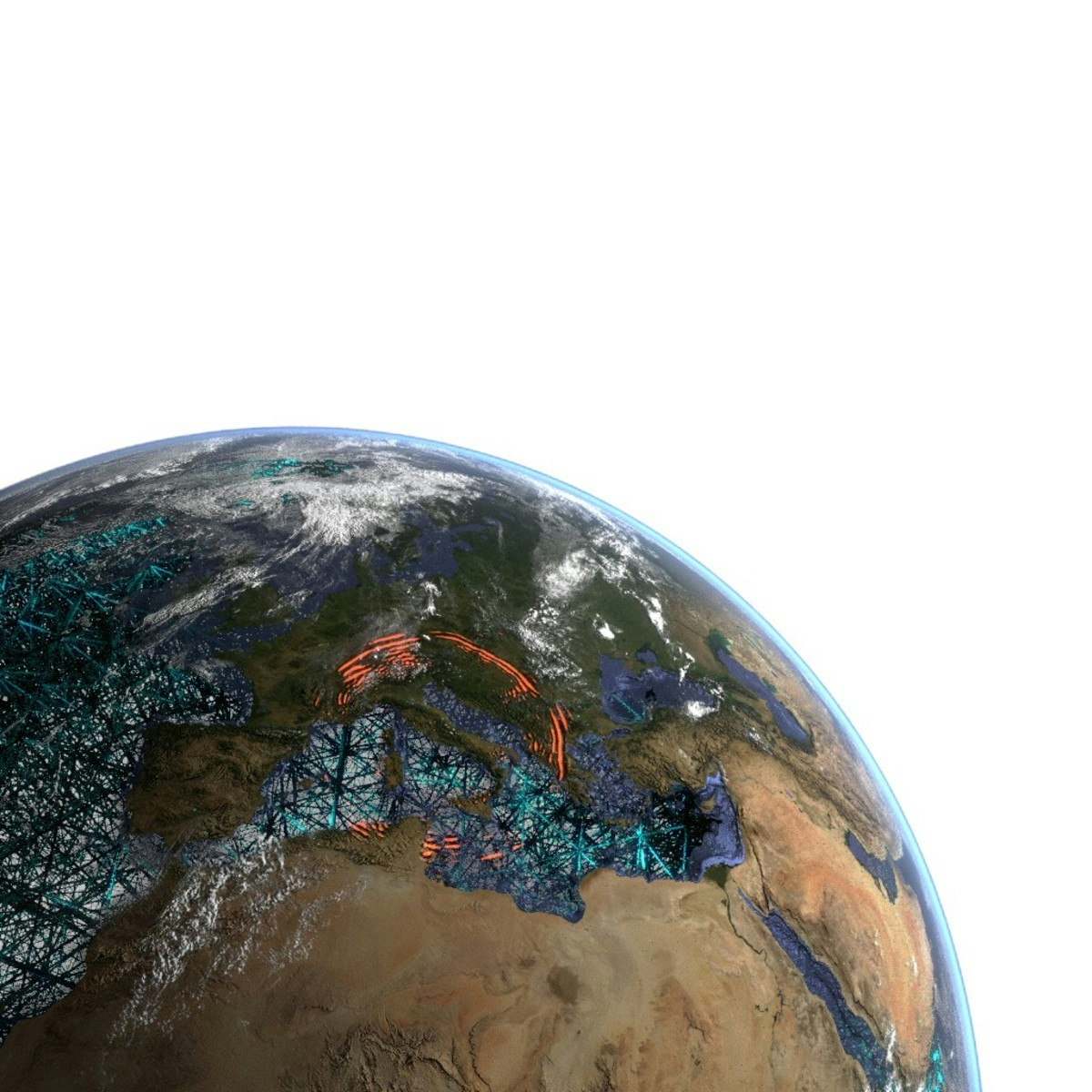>>

## Computers, Waves, Simulations: A Practical Introduction to Numerical Methods using Python

### University/Institute: Ludwig-Maximilians-Universität München LMU## Description

Interested in learning how to solve partial differential equations with numerical methods and how to turn them into python codes? This course provides you with a basic introduction how to apply methods like the finite-difference method, the pseudospectral method, the linear and spectral element method to the 1D (or 2D) scalar wave equation. The mathematical derivation of the computational algorithm is accompanied by python codes embedded in Jupyter notebooks. In a unique setup you can see how the mathematical equations are transformed to a computer code and the results visualized. The emphasis is on illustrating the fundamental mathematical ingredients of the various numerical methods (e.g, Taylor series, Fourier series, differentiation, function interpolation, numerical integration) and how they compare. You will be provided with strategies how to ensure your solutions are correct, for example benchmarking with analytical solutions or convergence tests. The mathematical aspects are complemented by a basic introduction to wave physics, discretization, meshes, parallel programming, computing models. The course targets anyone who aims at developing or using numerical methods applied to partial differential equations and is seeking a practical introduction at a basic level. The methodologies discussed are widely used in natural sciences, engineering, as well as economics and other fields.